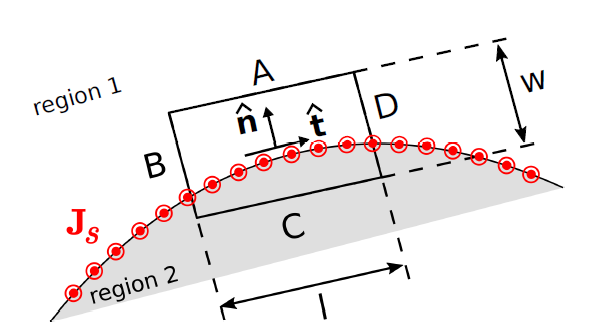# Boundary Conditions on the Magnetic Field Intensity (H)

In homogeneous media, electromagnetic quantities vary smoothly and continuously. At a boundary between dissimilar media, however, it is possible for electromagnetic quantities to be discontinuous. Continuities and discontinuities in fields can be described mathematically by boundary conditions and used to constrain solutions for fields away from these boundaries. In this section, we derive boundary conditions on the magnetic field intensity

.Figure 7.11.1: Determining the boundary condition on H at the smooth boundary between two material regions.

To begin, consider a region consisting of only two media that meet at a smooth boundary as shown in Figure 7.11.1. The desired boundary condition can be obtained directly from Ampere’s Circuital Law (ACL; Section 7.4):

where

is any closed path and

is the current that flows through the surface bounded by that path in the direction specified by the “right-hand rule” of Stokes’ theorem.

Let

take the form of a rectangle centered on a point on the boundary as shown in Figure 7.11.1, perpendicular to the direction of current flow at that location. Let the sides

,

,

, and

be perpendicular and parallel to the boundary. Let the length of the parallel sides be

, and let the length of the perpendicular sides be

. Now we apply ACL. We must integrate in a counter-clockwise direction in order to be consistent with the indicated reference direction for

. Thus:

Now we let

and

become vanishingly small while (1) maintaining the ratio

and (2) keeping

centered on the boundary. In this process, the contributions from the

and

segments become equal in magnitude but opposite in sign; i.e.,

This leaves

Let us define the unit vector

(“tangent”) as shown in Figure 7.11.1. Now we have simply:

where

and

are the fields evaluated on the two sides of the boundary, and

is the length of sides

and

. As always,

(units of A) may be interpreted as the flux of the current density

(units of A/m) flowing past a line on the surface having length

(units of m) perpendicular to

, where

is the normal to the surface, pointing into Region 1. Stated mathematically:

Before proceeding, note this is true regardless of the particular direction we selected for

; it is only necessary that

be tangent to the boundary. Thus,

need not necessarily be in the same direction as

. Now Equation 7.11.5 can be written:

Eliminating the common factor of

and arranging terms on the left:

The right side may be transformed using a vector identity (Equation 10.6.1 of Section 10.6) to obtain:

Equation 7.11.9 is the boundary condition we seek. We have found that the component of

(the difference between the magnetic field intensities at the boundary) in any direction tangent to the boundary is equal to the component of the current density flowing in the perpendicular direction

. Said differently:

A discontinuity in the tangential component of the magnetic field intensity at the boundary must be supported by surface current flowing in a direction perpendicular to this component of the field.

An important consequence is that:

If there is no surface current, then the tangential component of the magnetic field intensity is continuous across the boundary.

It is possible to obtain a mathematical form of the boundary condition that is more concise and often more useful than Equation 7.11.9. This form may be obtained as follows. First, we note that the dot product with respect to

on both sides of Equation 7.11.9 means simply “any component that is tangent to the boundary.” We need merely to make sure we are comparing the same tangential component on each side of the equation. For example

is tangential to the boundary, since

is perpendicular to the boundary and therefore any cross product involving

will be perpendicular to

. The corresponding component of the current density is

, so Equation 7.11.9 may be equivalently written as follows:

Applying a vector identity (Equation 10.6.1 of Section 10.6) to the right side of Equation 7.11.10 we obtain:

Therefore:

The minus sign on the right can be eliminated by swapping

and

on the left, yielding

This is the form in which the boundary condition is most commonly expressed.

It is worth noting what this means for the magnetic field intensity

. Since

:

In the absence of surface current, the tangential component of

across the boundary between two material regions is discontinuous if the permeabilities are unequal.

Ellingson, Steven W. (2018) Electromagnetics, Vol. 1. Blacksburg, VA: VT Publishing. https://doi.org/10.21061/electromagnetics-vol-1 CC BY-SA 4.0Swipe left and right to change pages.